• 主要为大家详细介绍了Python实现简单层次聚类算法以及可视化，具有一定的参考价值，感兴趣的小伙伴们可以参考一下
• 主要介绍了Python聚类算法之凝聚层次聚类的原理与具体使用技巧,具有一定参考借鉴价值,需要的朋友可以参考下
• 层次聚类算法 顾名思义，层次聚类就是一层一层的进行聚类，可以由上向下把大的类别（cluster）分割，叫作分裂法；也可以由下向上对小的类别进行聚合，叫作凝聚法；但是一般用的比较多的是由下向上的凝聚方法。 分裂...

层次聚类算法

顾名思义，层次聚类就是一层一层的进行聚类，可以由上向下把大的类别（cluster）分割，叫作分裂法；也可以由下向上对小的类别进行聚合，叫作凝聚法；但是一般用的比较多的是由下向上的凝聚方法。

分裂法：

分裂法指的是初始时将所有的样本归为一个类簇，然后依据某种准则进行逐渐的分裂，直到达到某种条件或者达到设定的分类数目。用算法描述：
输入：样本集合D，聚类数目或者某个条件（一般是样本距离的阈值，这样就可不设置聚类数目）
输出：聚类结果

1.将样本集中的所有的样本归为一个类簇；
repeat：
2.在同一个类簇（计为c）中计算两两样本之间的距离，找出距离最远的两个样本a,b；
3.将样本a，b分配到不同的类簇c1和c2中；
4.计算原类簇（c）中剩余的其他样本点和a，b的距离，若是dis(a)<dis(b)，则将样本点归到c1中，否则归到c2中；
util： 达到聚类的数目或者达到设定的条件

凝聚法：

凝聚法指的是初始时将每个样本点当做一个类簇，所以原始类簇的大小等于样本点的个数，然后依据某种准则合并这些初始的类簇，直到达到某种条件或者达到设定的分类数目。用算法描述：
输入：样本集合D，聚类数目或者某个条件（一般是样本距离的阈值，这样就可不设置聚类数目）
输出：聚类结果

1.将样本集中的所有的样本点都当做一个独立的类簇；
repeat：
2.计算两两类簇之间的距离（后边会做介绍），找到距离最小的两个类簇c1和c2；
3.合并类簇c1和c2为一个类簇；
util： 达到聚类的数目或者达到设定的条件

例图：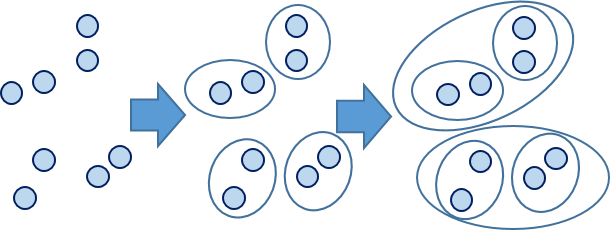类簇间距离的计算方法有许多种：
(1)就是取两个类中距离最近的两个样本的距离作为这两个集合的距离，也就是说，最近两个样本之间的距离越小，这两个类之间的相似度就越大
(2)取两个集合中距离最远的两个点的距离作为两个集合的距离
(3)把两个集合中的点两两的距离全部放在一起求一个平均值，相对也能得到合适一点的结果。
e.g.对于u中所有点i和v中所有点j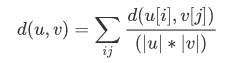其中|u|，|v|是聚类u和v中元素的的个数，这也被称为UPGMA算法（非加权组平均）法。
(4)取两两距离的中值，与取均值相比更加能够解除个别偏离样本对结果的干扰。
(5)求每个集合的中心点(就是将集合中的所有元素的对应维度相加然后再除以元素个数得到的一个向量)，然后用中心点代替集合再去就集合间的距离

实现

接下来以世界银行样本数据集进行简单实现。该数据集以标准格式存储在名为WBClust2013.csv的CSV格式的文件中。其有80行数据和14个变量。数据来源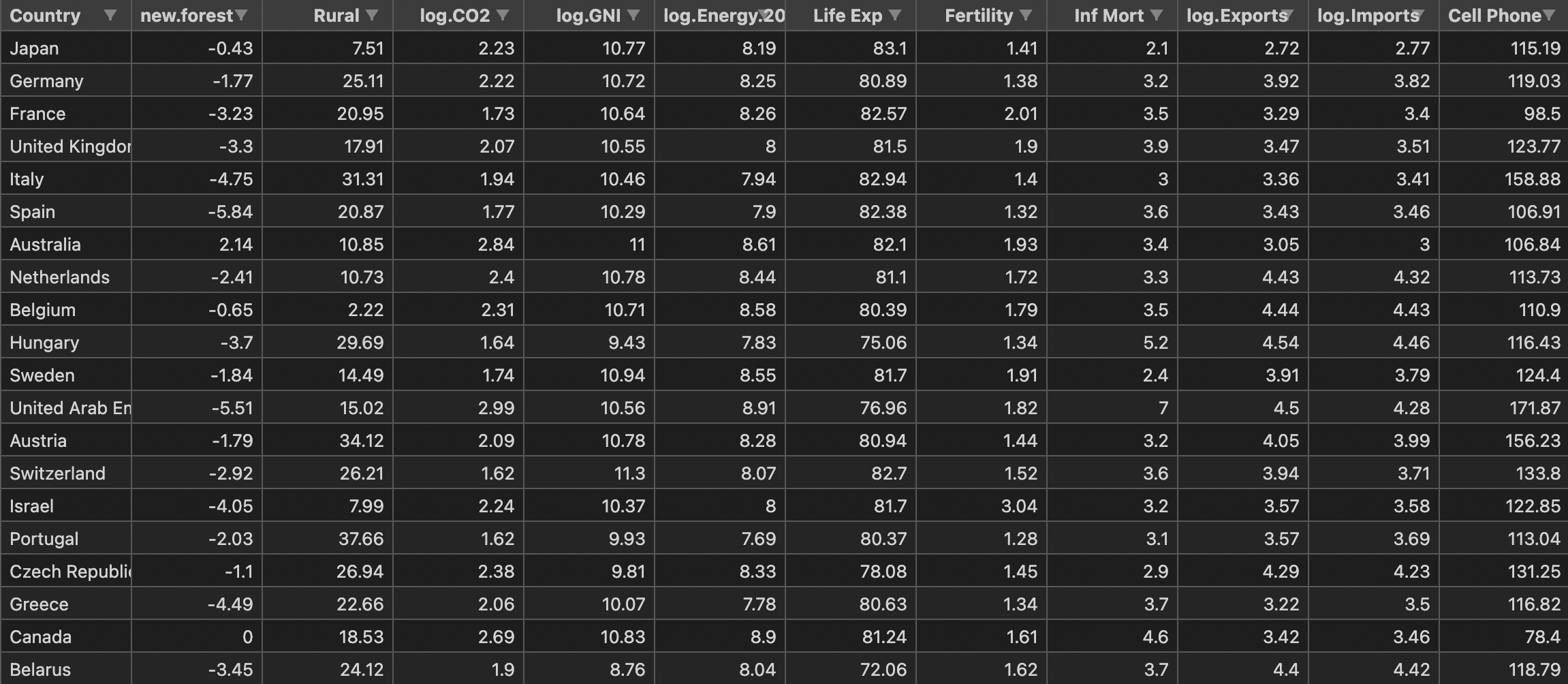将数据分为三个聚簇，并且在实现层次聚类之后加入PCA降维与原始结果进行对比。

from scipy.cluster.hierarchy import linkage, dendrogram, fcluster
import matplotlib.pyplot as plt
import pandas as pd
import numpy as np

data = data[:20]
country = list(data['Country'])
data.pop('Country')
data_zs = 1.0*data/data.max()#归一化
# print(data_zs)

# 以下代码为仅使用层次聚类
plt.figure(figsize=(9, 7))
plt.title("original data")
dendrogram(mergings, labels=country, leaf_rotation=45, leaf_font_size=8)
plt.show()

cluster_assignments = fcluster(mergings, t=3.0, criterion='maxclust')
print(cluster_assignments)
for i in range(1, 4):
print('cluster', i, ':')
num = 1
for index, value in enumerate(cluster_assignments):
if value == i:
if num % 5 == 0:
print()
num += 1
print(country[index], end='  ')
print()

# 以下代码为加入PCA进行对比
class myPCA():

def __init__(self, X, d=2):
self.X = X
self.d = d

def mean_center(self, data):
"""
去中心化
:param data: data sets
:return:
"""
n, m = data.shape
for i in range(m):
aver = np.sum(self.X[:, i])/n
x = np.tile(aver, (1, n))
self.X[:, i] = self.X[:, i]-x

def runPCA(self):

# 计算协方差矩阵，得到特征值，特征向量
S = np.dot(self.X.T, self.X)
S_val, S_victors = np.linalg.eig(S)
index = np.argsort(-S_val)[0:self.d]
Y = S_victors[:, index]
# 得到输出样本集
Y = np.dot(self.X, Y)
return Y

data_for_pca = np.array(data_zs)
pcaObject=myPCA(data_for_pca,d=2)
pcaObject.mean_center(data_for_pca)
res=pcaObject.runPCA()

# plt.figure(figsize=(9, 7))
# plt.title("after pca")
# print(mergings)
# dendrogram(mergings,labels=country,leaf_rotation=45,leaf_font_size=8)
# plt.show()

# cluster_assignments = fcluster(mergings, t=3.0, criterion='maxclust')
# print(cluster_assignments)
# for i in range(1,4):
#     print('cluster', i, ':')
#     num = 1
#     for index, value in enumerate(cluster_assignments):
#         if value == i:
#             if num % 5 ==0:
#                 print()
#             num+=1
#             print(country[index],end='  ')
#     print()

原始数据分类后的聚簇：

cluster 1 :
cluster 2 :
United States  Indonesia  Brazil  Russian Federation
Japan  Mexico  Germany  Turkey  Thailand
France  United Kingdom
cluster 3 :
China  India  Philippines  Vietnam
Egypt, Arab Rep.

PCA降维后的分类聚簇：

cluster 1 :
cluster 2 :
China  India  Philippines  Vietnam
Egypt, Arab Rep.
cluster 3 :
United States  Indonesia  Brazil  Russian Federation
Japan  Mexico  Germany  Turkey  Thailand
France  United Kingdom

可以看出，分类结果都是一样的。

通过树状图对结果进行可视化

以下为建树数据（以原始数据为例）：

[[18.         19.          0.21641882  2.        ]
[15.         20.          0.32365701  3.        ]
[ 2.          9.          0.39212766  2.        ]
[16.         21.          0.45344319  4.        ]
[ 8.         22.          0.50024778  3.        ]
[ 4.         10.          0.50648091  2.        ]
[13.         14.          0.51344362  2.        ]
[23.         24.          0.58762227  7.        ]
[ 3.         25.          0.58872577  3.        ]
[11.         26.          0.64184605  3.        ]
[ 5.          6.          0.71918707  2.        ]
[17.         28.          0.72310738  4.        ]
[ 0.          1.          0.84679303  2.        ]
[ 7.         12.          0.90714675  2.        ]
[30.         33.          0.97508395  4.        ]
[27.         31.          1.00112956 11.        ]
[29.         32.          1.15491503  5.        ]
[35.         36.          1.29675568 16.        ]
[34.         37.          1.76337101 20.        ]]

对以上数据解析为：第一列和第二列为聚类簇编号；第三列为两个聚簇之间的距离；第四列为包含的聚簇数量。
其中聚簇间距离的计算为上文提到的第三种方法。

原始数据树状图：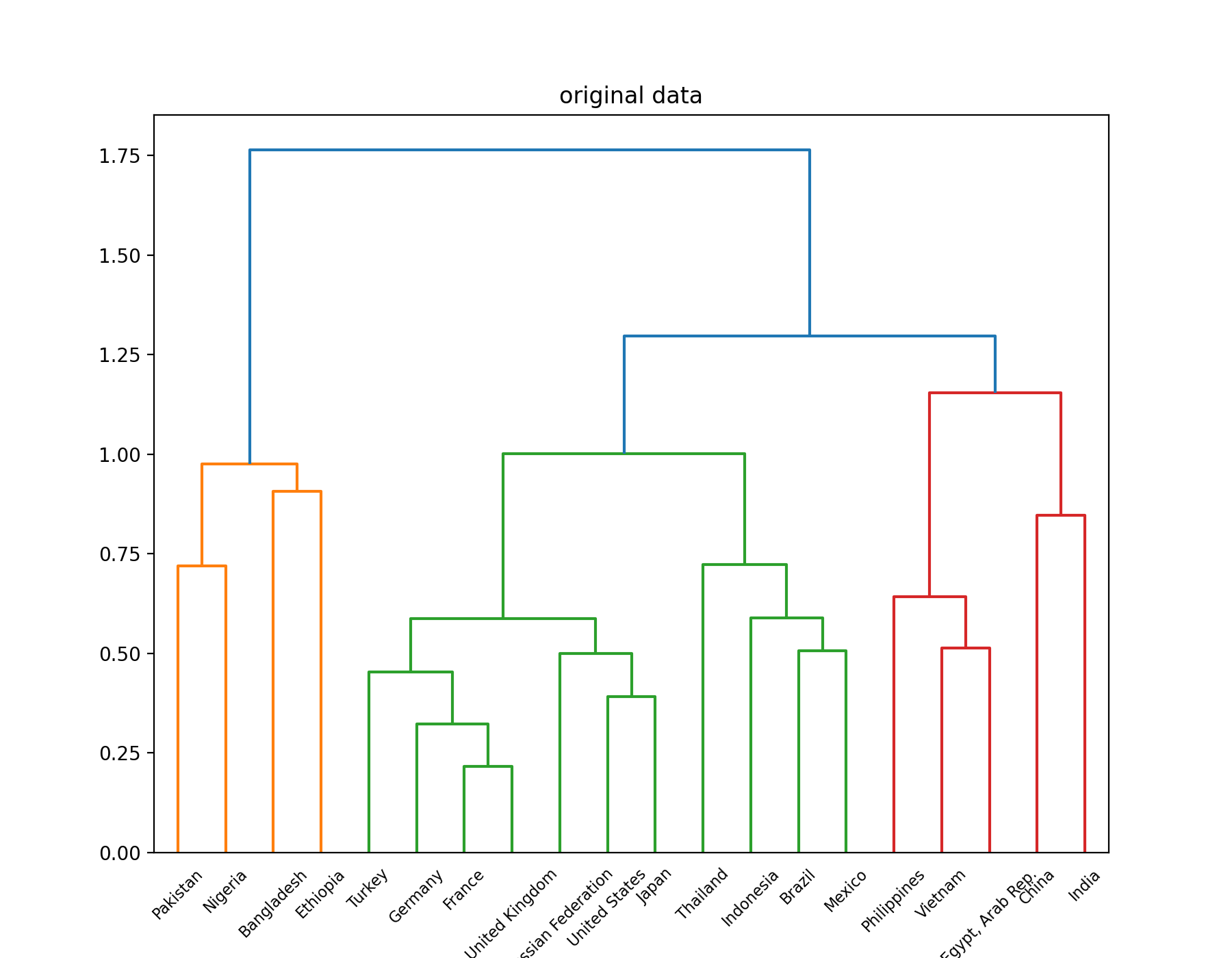PCA降维后的结果：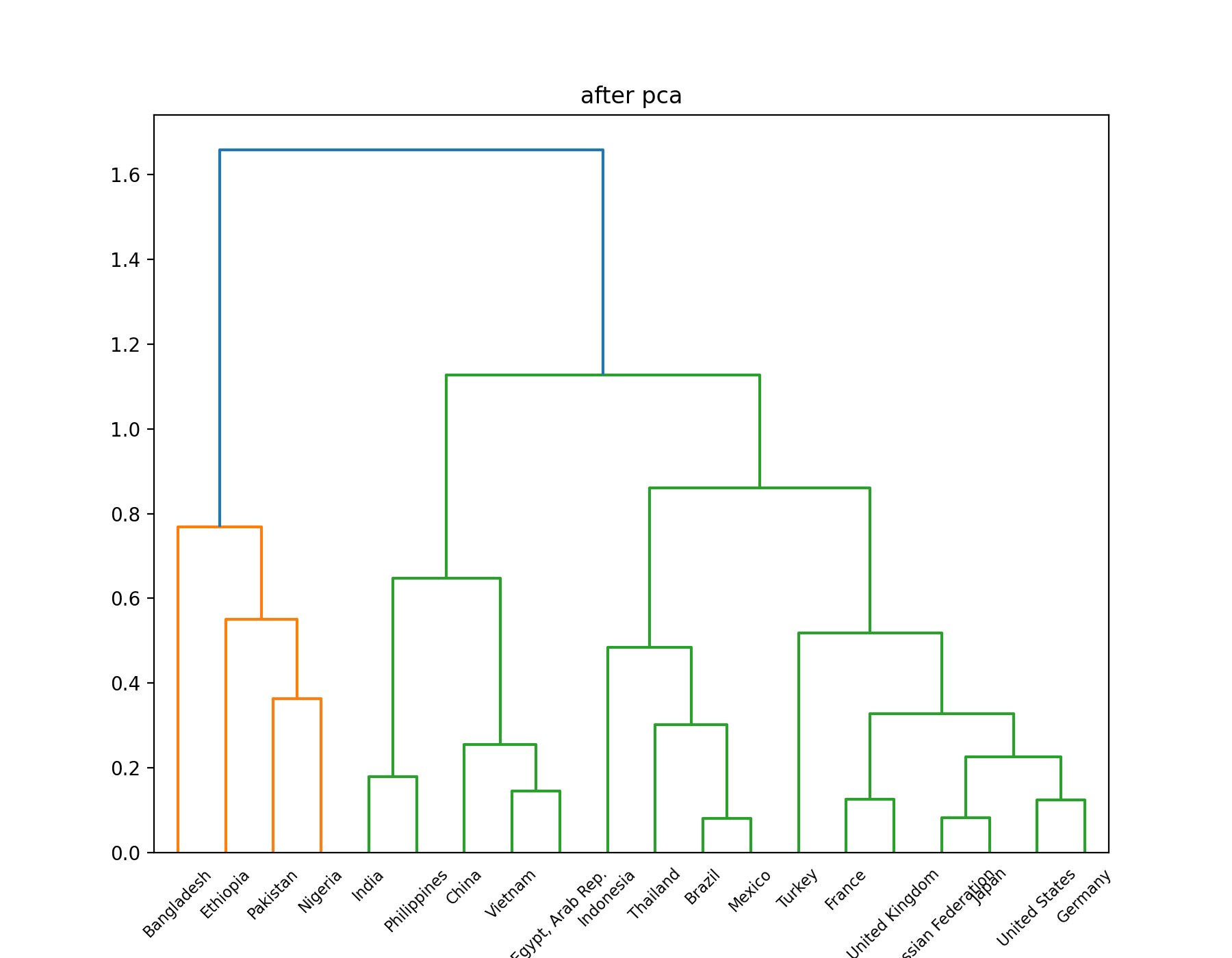展开全文聚类 python 机器学习
• 这个是一个简单的聚类分析matlab代码实现，通过matlab对数据进行了简单的层次聚类分析matlab
• 包括最长、最短欧式距离法、重心法（标准欧式、平方欧式、精度加权）、平均法、权重法等等
• 层次聚类代码

2018-05-02 16:26:22
自己做的层次聚类 有可视化 有数据集 基于熵特征的聚类
• 层次聚类matlab代码，数据要求字符串格式，数据类型一致，便于计算和使用，提高数据准确度和可用性，简单实用。层次聚类
• 前言聚类分析是研究分类问题的分析方法，是洞察用户偏好和做用户画像的利器之一。...本文将详细介绍如何 利用 Python 实现基于层次聚类的客户分群，主要分为两个部分：层次聚类详细原理介绍Python ...

前言

聚类分析是研究分类问题的分析方法，是洞察用户偏好和做用户画像的利器之一。聚类分析的方法非常多，能够理解经典又最基础的聚类方法 —— 层次聚类法(系统聚类) 的基本原理并将代码用于实际的业务案例是本文的目标，同时这也会为理解后续与聚类相关的推文如 K-Means 等打下基础是。

本文将详细介绍如何 利用 Python 实现基于层次聚类的客户分群，主要分为两个部分：

层次聚类详细原理介绍

Python 代码实战讲解

原理部分

原理介绍

既然它们能被看成是一类的，所以要么它们距离近，要么它们或多或少有共同的特征。拿到数据集后，直接根据特征或指标来将样本分类的做法其实更适合业务能力比较强的人或有了十分明确的指标如男女各一类等硬性要求，所以本文以样本之间的距离为聚类指标。为了能够更好地深入浅出，我们调整了一下学习顺序，将小部分数学公式往后放，先从聚类结果的显示与分析入手。

下面是有关层次聚类的几个常见问题。

1、为什么都说层次树是层次聚类法独有的聚类结果图？

因为树形图的横坐标会将每一个样本都标出来，并展示聚类的过程。几十个样本时候层次树就已经 “无法” 查看了，更何况成百上千的数据样本。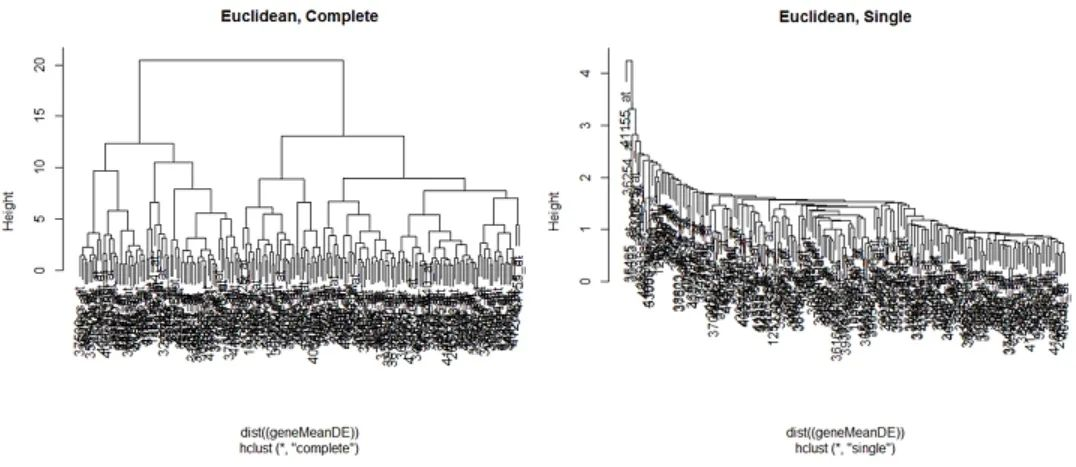2、层次树是怎么建立的？建立的基本步骤？

其实层次树的建立过程表示的就是聚类的过程，只不过通过层次树我们可以看出类之间的层次关系(这一类与那一类相差多远)，同时还可以通过层次树决定最佳的聚类个数和看出聚类方式(聚类顺序的先后)

基本步骤比较简洁，只要短短的 3 步：

计算每两个观测之间的距离

将最近的两个观测聚为一类，将其看作一个整体计算与其它观测(类)之间的距离

一直重复上述过程，直至所有的观测被聚为一类

建立层次树的三个步骤虽然简洁，但其实也有令人迷惑的地方，所以为了让各位更好的从整体上去理解聚类过程而不是圄于细节，这里先直接放一个聚类过程图和对应的层次树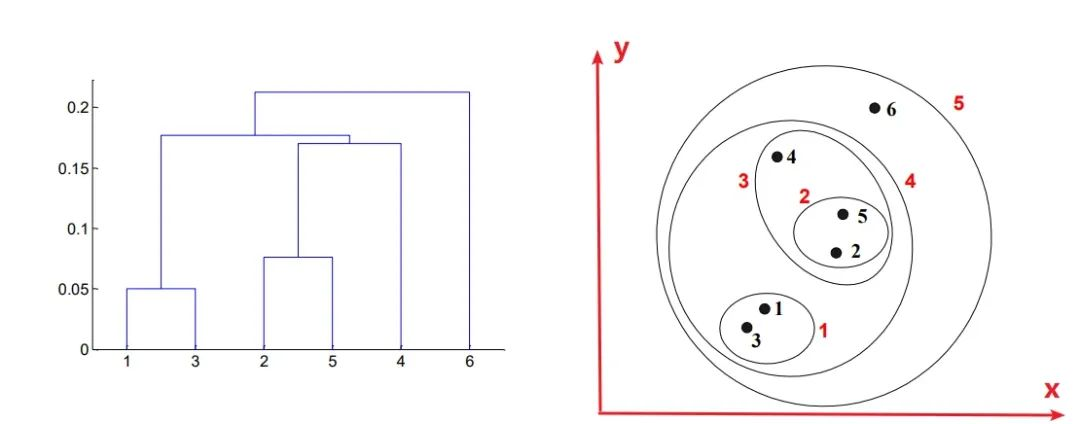3、怎么从层次树中看出聚类过程？

这一个简短的问题中其实暗含不少门道，第一：**当两个点被分为一类时，是从横坐标出发向上延伸，后形成一条横杠；当两个类被分为一类时，是横杠中点向上延伸。**这第一点中，横杠的数量就表示当所有的点都被圈为一类的时候经过了多少次聚类。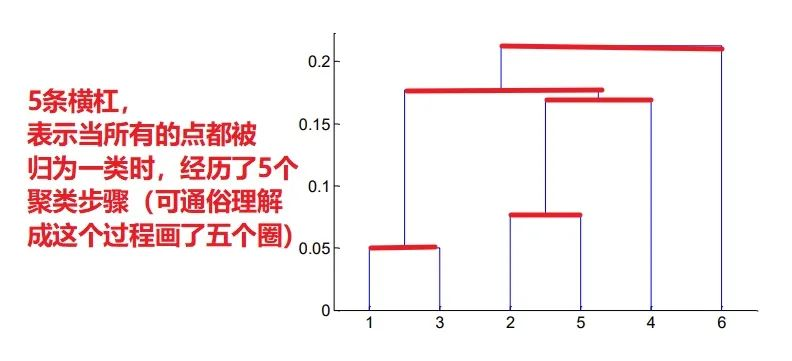同样，横杠距离横坐标轴的高度也有玄机，毕竟每生成一个横杠就表示又有一次聚类了，所以我们可以通过横杠的高度判断聚类的顺序，结合上图右半部分的圆圈和数字标号也可以看出。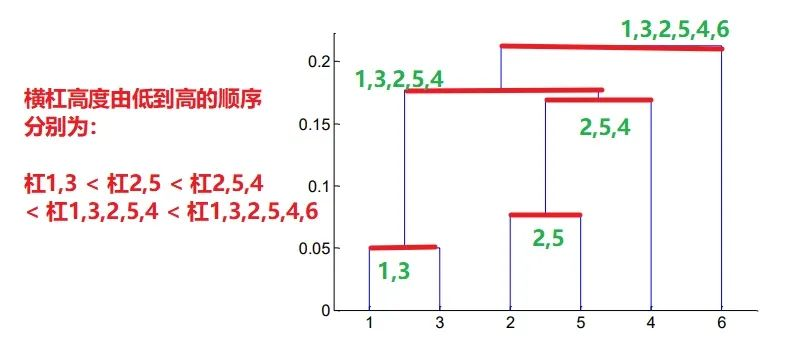所以聚类顺序便如下表：

聚类次数

被聚为一类的点

第1次

1和3     →    1,3

第2次

2和5     →     2,5

第3次

2,5 和 4   →   2,5,4

第4次

2,5,4 和 1,3   →   1,3,2,5,4

第5次

1,3,2,5,4 和 6   →   1,3,2,5,4,6(所有点被聚为一类)

第二，整棵层次树是由一棵棵小树组成，每棵小树代表了一个类，小树的高度即是两个点或两个类之间的距离，所以两个点之间的距离越近，这棵树就越矮小。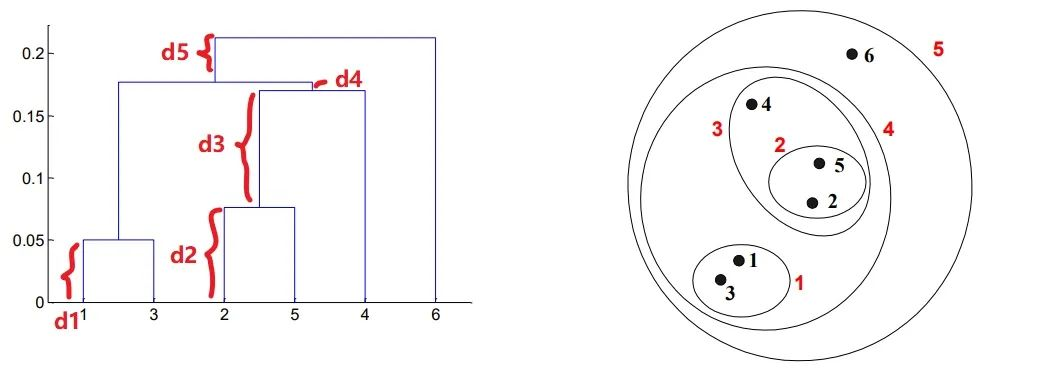下面这一段仔细阅读的话对理解点与点，类与类，点与类之间的距离是如何在层次树上体现很有帮助。先从最矮的高度只有 d1 小树说起，这就是类 1,3 中两个孤立的点 1 和 3 之间的距离；同理，d2 为类2,5 中点 2 和 5 之间的距离。

而至于 d3, d4, d5 这三个距离，他们并不像 d1 和 d2 那般表示的是一棵完整的树的高度，而更像是 “ 生长的枝干 ”，因为从第一点中的 “ 当两个类被分为一类时，是横杠中点向上延伸。” 可以看出 d3 是从类 2,5 横杠的中点往上延伸的，所以它表示会与另外的类聚成一起并形成一棵更大的树，图中即类 2,5 和点 4 被聚成一个新的类 2,5,4。

同理：

d4 表示类 2,5,4 与类 1,3 聚成新类 1,3,2,5,4

d5 表示类 1,3,2,5,4 与点 6 聚成类 1,3,2,5,4,6

4、怎么从层次树中看出聚类情况？可以通过决定纵轴分界线可决定这些数据到底是分成多少类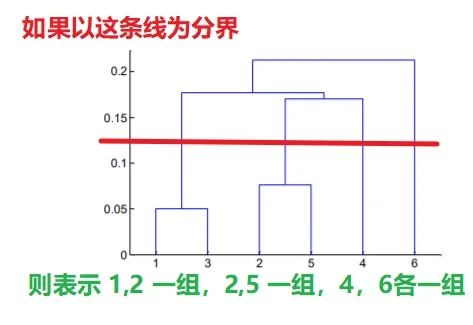定好分界线后，只需要看距离这条线横杠和单独的竖线即可，上图中距离红线的横杠有两条(分别表示类1,2 和类2,5)，单独的竖线也有两条，从横坐标轴 4 和 6 上各延伸出的一条。同理可用到下图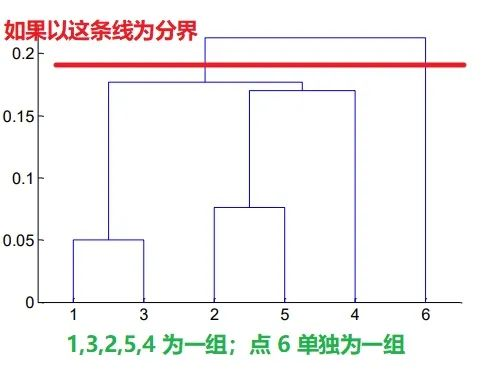为什么最好不要分成 3 组：13，254，6 呢？

因为树的高度表示两个点之间的距离，所以 4 到 类25 的距离只比到 类13 的距离要多如下图所示的一点点，所以硬是把 4 跟 25 分成一类就有点牵强了，正因为这种牵强的分类方式可能会让我们忽略 4 这个点单独的价值，所以我们不如直接将 4 看成单独的一类。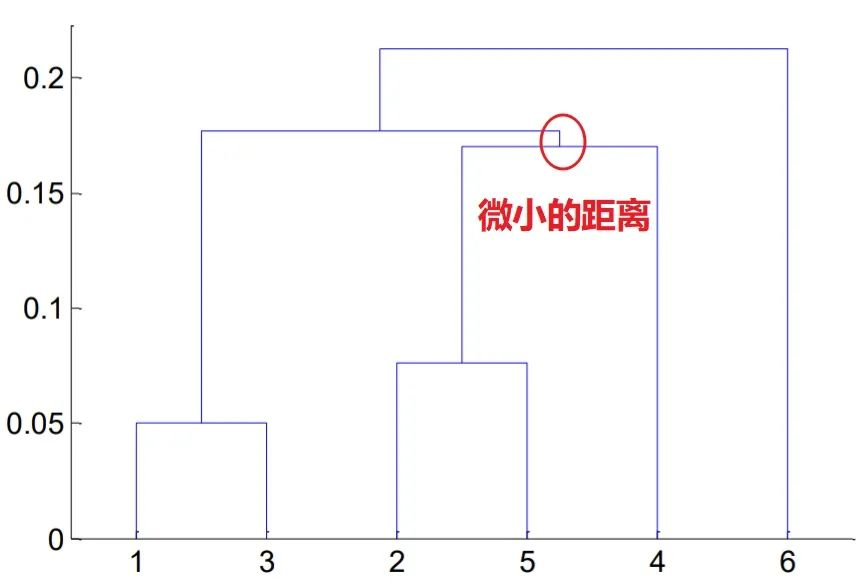推导与计算

接下来就是需要更加动脑的数学原理和公式部分了，我们需要知晓点与点，类与类，点与类这三种距离如何计算。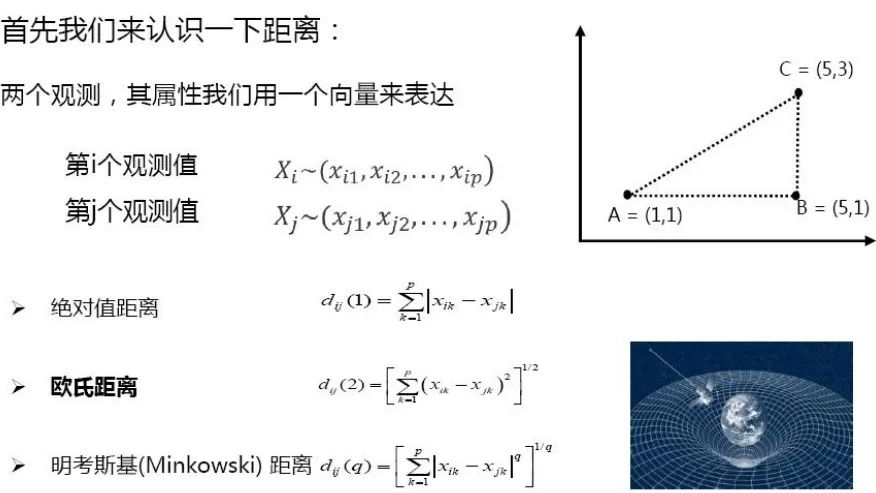最包罗万象的是明考斯基距离，因为 q 分别取 1 和 2 的时候就表示是绝对值距离和欧氏距离。点与点的距离很好求，我们一般用的都是欧氏距离，即初中学习的直角三角形三边关系，上图右上角点AC之间的距离(ab² + bc²) 后再开根号

而至于类与类之间的距离求法，其实经过了一个演变，篇幅原因本文只会一笔带过那些不常用的方法并将重心放在最常用和主流的方法上。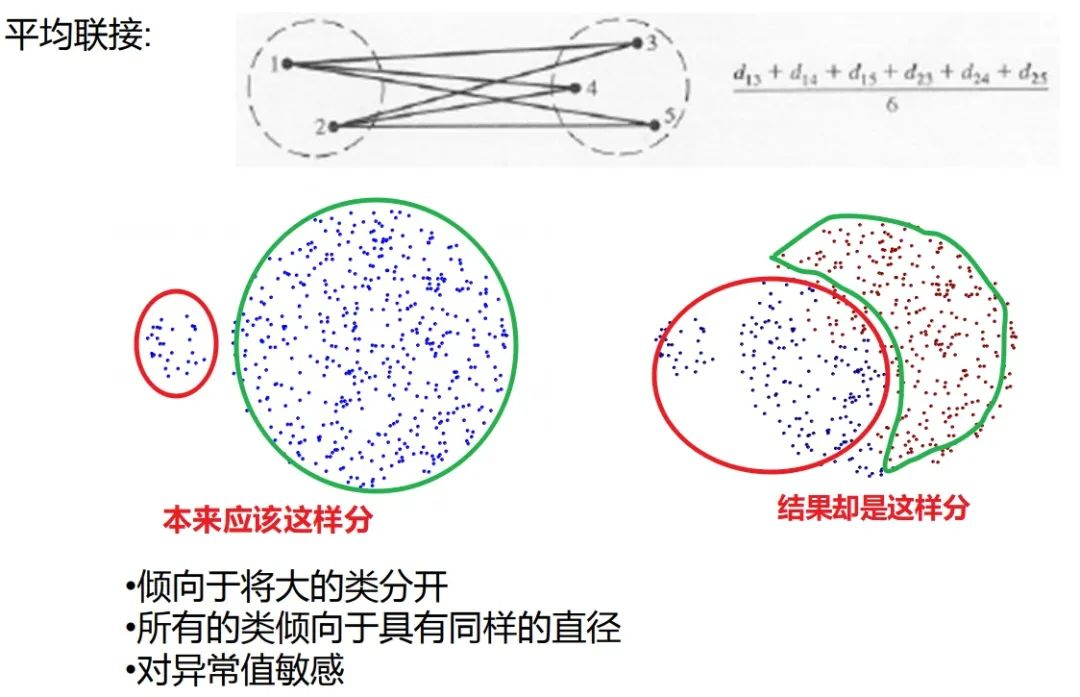平均联接和重心法都已经比较少用了，现大多采用较少受异常值影响且不知数据分布的情况下依然能有较好表现的 Ward 法。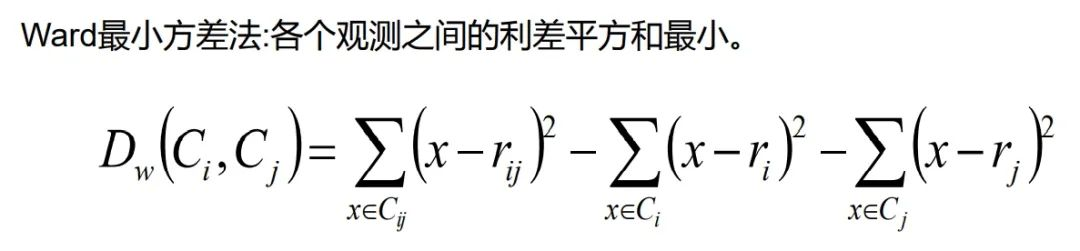其实 Ward 法的公式与方差分析相似，都是通过组间距离来定夺点点/点类/类类间的距离，Ward 法许多详细的数学推导在网上有很多，这里我们直接展示最容易理解的一种：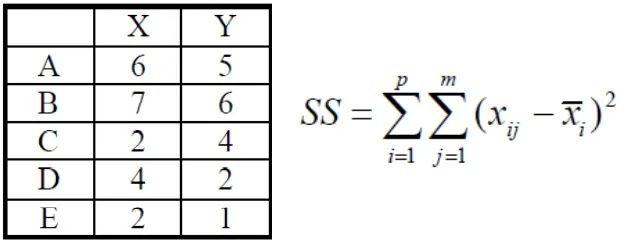上图为已知的五个点和它们 x，y 轴坐标，SS 为 Ward 最小方差法的公式。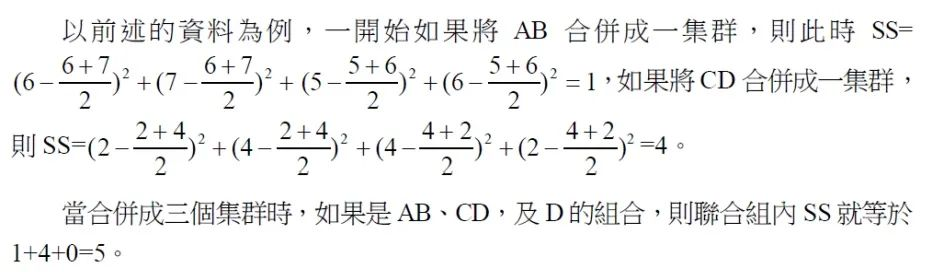当两个点被聚为一类时，这一类的组间差异 SS 便为其中一点的某一坐标与另外的所有坐标加加减减的一系列操作(通俗解释，其实直接看上图的 SS 计算过程已经可以理解。)

了解 Ward 最小方差法的基本求解公式后，我们可以从最简单的聚类形式开始：5个点聚成4类。这意味着其中两个点会被聚在一起，剩下三个点各自为一类，所以总共会出现 C52 = 10 中情况，每种情况的组内 SS 分别如下表：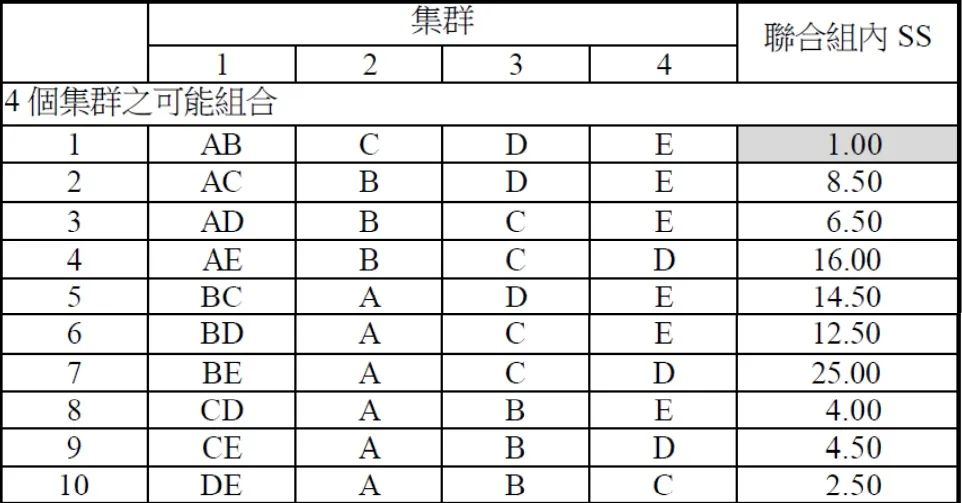同理，如果这 5 个点聚成 3/2/1 类的情况如下表：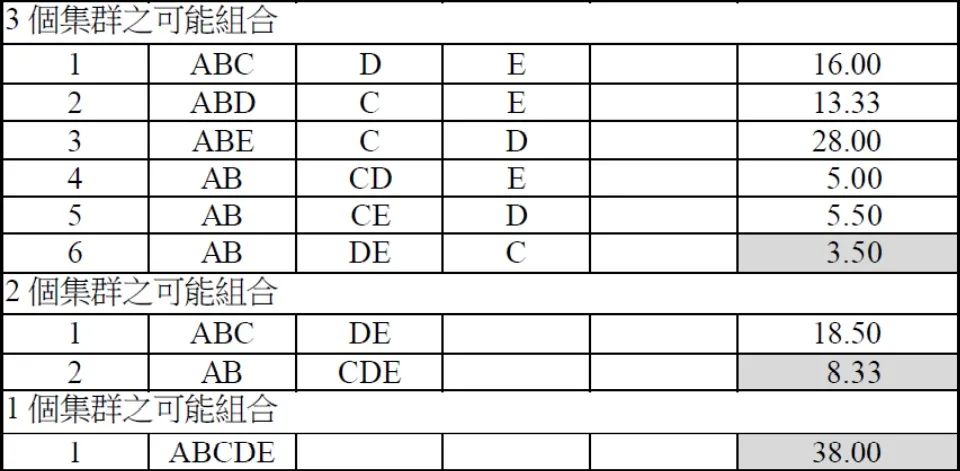需要注意的是：聚成两类后计算出 AB 这两个的利方差最小后，在后续聚成3类，2类的时候就直接把A和B两个看成是同一个个体了，所以不会再出现A和B分开的局面。

结合两个表，我们便可以得出如下结论：

如果需要被

聚成 4 类，AB为一类，剩下3个点各为一类最好(SS 最小)

如果需要被

聚成 3 类，AB，DE为一类，剩下的 C 单独为一类最好

如果需要被

聚成 2 类，AB，CDE各位一类

如果需要被

聚成 1 类，对不起，我觉得没什么分析的必要

在进入代码实战前，我们简单总结一下原理部分提到的知识点：

层次树的阅读

两个点之间的距离公式

Ward 法求类内的组间差异，用以决定聚出的类别个数

代码实战

在正式实战前，需要注意以下几点，首先原始数据通常需要经过处理才能用于分析：

缺失值

异常值(极大或极小)

分类变量需要转化为哑变量(0/1数值)

分类变量类别不宜过多

其次由于变量的量纲的不一样引起计算距离的偏差，我们需要对数据进行标准化。同时不同的统计方法对数据有不同的要求：

决策树和随机森林允许缺失值和异常值

聚类分析和回归模型则不支持缺失值

在处理数据时，也有两个问题值得关注，

1、聚类的时候，所有的 X 必须都是连续变量，为什么？

分类变量无法计算距离，如某个变量表示的是性别，男和女；教育程度为小学，初中，高中，大学，那该变量在各个个体之间的距离怎么计算？所以做聚类分析时尽可能用分类变量。

2、那这些分类变量的价值难道就无法利用了吗？

可以先根据其他的连续变量聚类，而后对分出来的类做描述性统计分析，这时候就可以用上分类变量的价值了。另外一种方法是可以在第一步就把分类变量也用上的聚类方法，不过需要结合实际业务。

以市场客户调研为例，属于 “ 客户的需求与态度 ” 这个分支，目的是依据调查问卷结果针对需求的数据分群，而调查分卷的问题中回答 “yes” 或者 “no” 类型的问题通常又会占一大部分，这时候我么可以通过合并多个问题回答的结果来将多个分类变量组合，生成一个连续变量，以电信客户的使用和需求情况为例：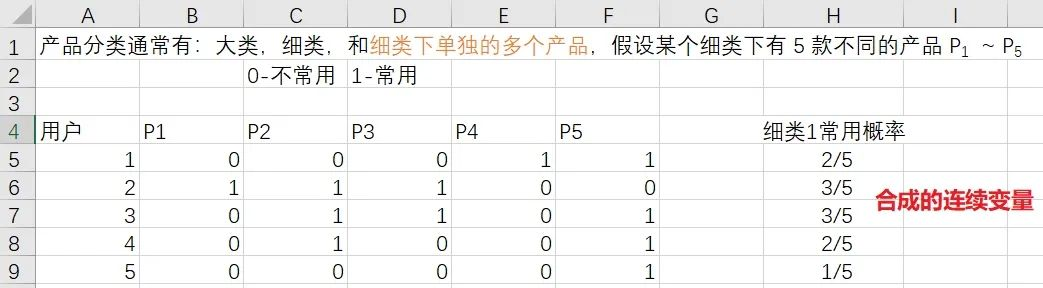当然也还可以计算分类变量之间的 cos 相似度，即直接把分类变量设成距离。总之，分类变量在聚类当中是一定需要处理的。

现在终于到了正式的代码阶段，如果前面的原理都理解好了，代码的理解则可不费吹灰之力。这里我们使用一份公开的城市经济数据集，参数如下：

AREA：城市名称

Gross：总体经济情况指数

Avg：平均经济情况指数

import pandas as pd

import numpy as np

import matplotlib.pyplot as plt

plt.rc('font', **{'family': 'Microsoft YaHei, SimHei'})  # 设置中文字体的支持

plt.rcParams['axes.unicode_minus'] = False

# 解决保存图像是负号'-'显示为方块的问题

df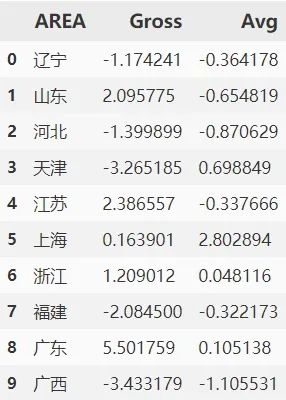这些城市的指标分布如下波士顿矩阵图，篇幅原因绘图代码省略，后台回复关键字获取的源程序会一并提供。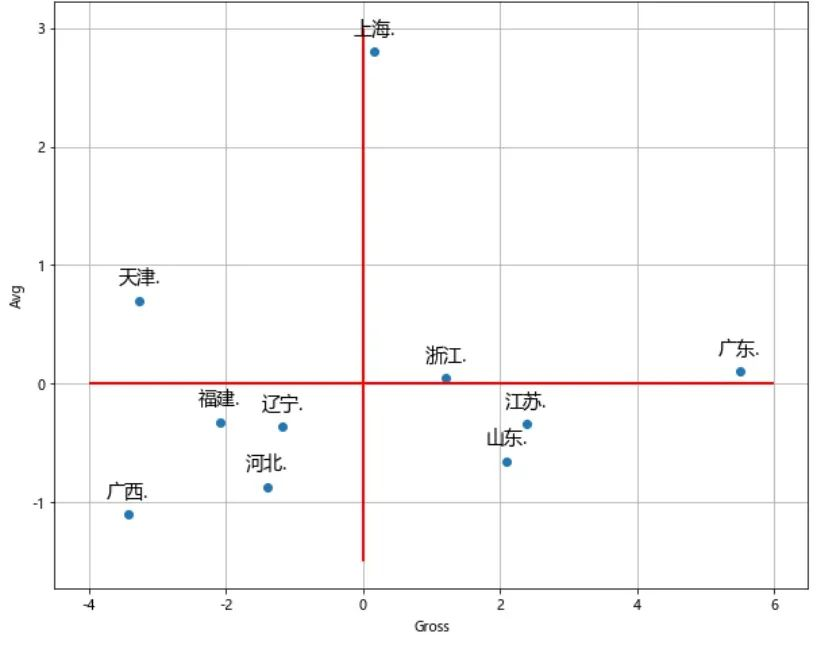sklearn 里面没有层次聚类的函数，所以从 scipy 中导入

import scipy.cluster.hierarchy as sch

# 生成点与点之间的距离矩阵, 这里用的欧氏距离: euclidean

## X：根据什么来聚类，这里结合总体情况 Gross 与平均情况 Avg 两者

disMat = sch.distance.pdist(X=df[['Gross', 'Avg']], metric='euclidean')

# 进行层次聚类: 计算距离的方法使用 ward 法

下面是层次聚类可视化：层次树

# 将层级聚类结果以树状图表示出来并保存

# 需要手动添加标签。

P = sch.dendrogram(Z, labels=df.AREA.tolist())

plt.savefig('聚类结果.png')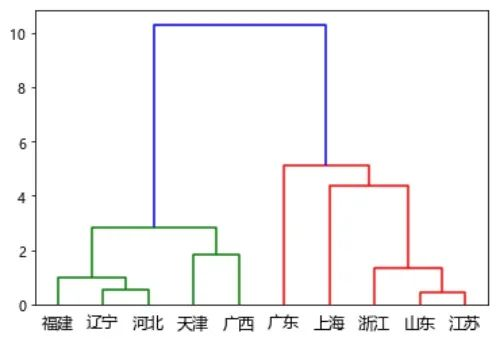最后说一下，未来还会有 K-Means 等聚类方法的推文。作为深入浅出聚类方法的开端，我们只需知道层次聚类相比 K-Means 的好处是它不用事先指定我们需要聚成几类 (K-Means 算法代码中的参数 k 指定)

这样一来，我们只需要把计算全权交给程序，最终能得出一个比较精准的结果。但缺点是随之而来的计算复杂度的增加。

所以层次聚类更适合小样本；K-Means 更适合大样本，在数据挖掘中也更常见，本文分享就到这里，我们下期决策树见～

展开全文• 该算法是自底而上的算法，他的主要原理是所有数据每个样本看成一个初始聚类，在算法运行过程中不断找出距离自己最近的聚类之后合并，直到达到自己设定的聚类个数，伪代码如下： C++代码如下 //DataPoint.h 保存基础...

C++实现AGNES算法

该算法是自底而上的算法，他的主要原理是所有数据每个样本看成一个初始聚类，在算法运行过程中不断找出距离自己最近的聚类之后合并，直到达到自己设定的聚类个数，所用数据集为python的鸢尾花数据，伪代码如下：C++代码如下

//DataPoint.h 保存基础数据的类
#ifndef _DATAPOINT_H_
#define  _DATAPOINT_H_

#include <vector>
#include <string>
#include <iostream>
#include<map>
using namespace std;
class DataPoint
{
public:
DataPoint() {}
~DataPoint() {}

vector<double> GetData(); //获取保存数据
string  GetName(); //获取花卉名字
int GetClusterId(); //获取聚类id

void SetClusterId(int number);//设置聚类id
void SetName(string str);//设置花卉名字
void SetData(vector<double> v);//设置保存数据
private:

vector<double> Data; //保存数据
string name; //花卉名字
int clusterId;//聚类id

};
#endif // _DATAPOINT_H_

//DataPoint.cpp
#include "DataPoint.h"
void DataPoint::SetClusterId(int number)
{
this->clusterId = number;
}
void DataPoint::SetData(vector<double> v)
{
for (int i = 0; i < v.size(); i++)
{
Data.push_back(v[i]);
}
}
void DataPoint::SetName(string name)
{
this->name = name;
}
vector<double> DataPoint::GetData()
{
return this->Data;
}
string DataPoint::GetName()
{
return this->name;
}
int DataPoint::GetClusterId()
{
return this->clusterId;
}

//AGNES.cpp
#ifndef _AGNES_H_
#define  _AGNES_H_

#include <iostream>
#include<string>
#include<vector>
#include <fstream>
#include <sstream>
#include "DataPoint.h"
using namespace std;
//将string数据转为数字
template <class Type>
Type stringToNum(const string& str)
{
istringstream iss(str);
Type num;
iss >> num;
return num;
}
class AGNES
{
public:
AGNES(double ThreshordDis, int MaxClu) :ThreshordDis(ThreshordDis), MaxClu(MaxClu) {}
~AGNES() {}
void StartAgnes(); //算法核心
void GetData();//获取数据
void print();//打印信息
private:
double GetDistance(DataPoint &point, DataPoint &point2); //得到两个数据之间的距离
double GetCDistance(vector<DataPoint> C, vector<DataPoint> C2); //得到两个聚类之间的最小距离
double GetAVGDistance(vector<DataPoint> C, vector<DataPoint> C2);//得到两个聚类之间的平均距离
vector<DataPoint> DataBase; //保存所有数据
vector<vector<DataPoint>> CluData; //保存所有簇类数据

double ThreshordDis; //这里暂时无用，可以省略
int MaxClu; //最终获得聚类数量
int  p; //存储最初的聚类的个数
};
#endif

//AGNES.cpp
#include "AGNES.h"
static double DataMap; //保存两两聚类之间的距离
void AGNES::GetData()
{
ifstream file;
string line;
file.open("iris.csv",ios::in);
if (file.fail())
{
cout << "文件打开失败" << endl;
}
while (getline(file, line))
{
stringstream ss(line);
string str;
vector<string> temp;
vector<double> v;
DataPoint d;
while (getline(ss, str, ','))
{
temp.push_back(str);
}
for (int i = 1; i < temp.size()-1; i++)
{
v.push_back(stringToNum<double>(temp[i]));
}
//初始化数据
d.SetData(v);
d.SetName(temp[temp.size() - 1]);
d.SetClusterId(stringToNum<int>(temp)-1);//因为数据是从1开始编号的所以这里-1使其从0开始编号
DataBase.push_back(d);
}
}

void AGNES::StartAgnes()
{
//获得每个数据距离其他数据的距离
for (int i = 0; i < DataBase.size(); i++)
{
vector<DataPoint> t;
t.push_back(DataBase[i]);
CluData.push_back(t);
}
//DataMap数组保存两个聚类之间的距离
for (int i = 0; i < CluData.size(); i++)
{
vector<DataPoint> temp;
for (int j =i+1; j < CluData.size(); j++)
{
double dis = GetAVGDistance(CluData[i], CluData[j]);
DataMap[i][j] = dis;
DataMap[j][i] = dis;
}
}
p = DataBase.size(); //设置聚类个数
while (p > MaxClu)
{
double Temp = 9999;
int Find_i = 0, Find_j = 0;
//寻找最小值点
for (int i = 0; i < p; i++)
{
for (int j = i+1; j < p; j++)
{
if (DataMap[i][j] < Temp)
{
Temp = DataMap[i][j];
Find_i = i;
Find_j = j;
}
}
}
int NewId = CluData[Find_i].GetClusterId(); //获取当前簇的聚类号码
//将寻找到的最小点的两个簇合并 A1+B1->A1
//并将两个簇的簇类号码统一
for (int j = 0; j < CluData[Find_j].size(); j++)
{
CluData[Find_j][j].SetClusterId(NewId);
CluData[Find_i].push_back(CluData[Find_j][j]);
}
//重编号矩阵
for (int i = Find_j + 1; i < p; i++)
{
for (int j = 0; j < CluData[i].size(); j++)
{
CluData[i][j].SetClusterId(CluData[i][j].GetClusterId() - 1);
}
}
//距离矩阵重置
for (int i = Find_j; i < CluData.size()-1; i++)
{
CluData[i] = CluData[i + 1];
}
//删除DataMap矩阵 行列
for (int i = 0; i < p; i++)
{
for (int j = Find_j; j < p-1; j++)
{
DataMap[i][j] = DataMap[i][j + 1];
}
}
for (int i = Find_j; i < p; i++)
{
for (int j = 0; j < p - 1; j++)
{
DataMap[i][j] = DataMap[i + 1][j];
}
}
p = p - 1;
//重新计算合并数据后的聚类与其他聚类之间的距离
for (int i = 0; i < p; ++i)
{
double dis = GetAVGDistance(CluData[Find_i], CluData[i]);
if (DataMap[Find_i][i] != dis)
{
DataMap[Find_i][i] = dis;
DataMap[i][Find_i] = dis;
}
}
}
}
//打印数据
void AGNES::print()
{
for (int i = 0; i < p; i++)
{
cout << "聚类编号：" << i << endl;
for (int j = 0; j < CluData[i].size(); j++)
{
vector<double> dat = CluData[i][j].GetData();
for (int s = 0; s < dat.size(); s++)
{
cout << dat[s] << " ";
}
cout << CluData[i][j].GetName() << " ";
cout << CluData[i][j].GetClusterId();
cout << endl;
}
}
}
//两个数据之间的距离
double AGNES::GetDistance(DataPoint &point, DataPoint &point2)
{
double sum = 0;
for (int i = 0; i < point.GetData().size(); i++)
{
sum += (point.GetData()[i] - point2.GetData()[i])*(point.GetData()[i] - point2.GetData()[i]);
}
double result = sqrt(sum);
return result;
}
//两个聚类之间最小的距离
double AGNES::GetCDistance(vector<DataPoint> C, vector<DataPoint> C2)
{
double MinDis = 9999;
for (int i = 0; i < C.size(); i++)
{
for (int j = 0; j < C2.size(); j++)
{
double dis = GetDistance(C[i], C2[j]);
if (dis < MinDis)
{
MinDis = dis;
}
}
}
return MinDis;
}
//两个聚类的平均距离
double AGNES::GetAVGDistance(vector<DataPoint> C, vector<DataPoint> C2)
{
double temp;
double temp2;
for (int i = 0; i < C.size(); i++)
{
temp += C[i].GetData();
temp += C[i].GetData();
temp += C[i].GetData();
temp += C[i].GetData();
}
temp = temp / C.size();
temp = temp / C.size();
temp = temp / C.size();
temp = temp / C.size();

for (int i = 0; i < C2.size(); i++)
{
temp2 += C2[i].GetData();
temp2 += C2[i].GetData();
temp2 += C2[i].GetData();
temp2 += C2[i].GetData();
}
temp2 = temp2 / C2.size();
temp2 = temp2 / C2.size();
temp2 = temp2 / C2.size();
temp2 = temp2 / C2.size();
int sum = 0;
for (int i = 0; i < 4; i++)
{
sum += ((temp[i] - temp2[i])*(temp[i] - temp2[i]));
}
return sqrt(sum);
}

展开全文机器学习 聚类算法 c++ 算法
• 层次聚类1.1 凝聚聚类1.2 层次图1.3 不同凝聚算法比较2. Sklearn 实现2.1 层次图可视化参考文献 1. 层次聚类 层次聚类（hierarchical clustering）试图在不同层次对数据集进行划分，从而形成树形的聚类结构。数据...

文章目录

相关文章：

机器学习 | 目录

机器学习 | 聚类评估指标

机器学习 | 距离计算

无监督学习 | KMeans 与 KMeans++ 原理

无监督学习 | DBSCAN 原理及Sklearn实现

无监督学习 | GMM 高斯混合聚类原理及Sklearn实现

1. 层次聚类

层次聚类（hierarchical clustering）试图在不同层次对数据集进行划分，从而形成树形的聚类结构。数据集的划分可采用“自底向上”的聚合策略，也可采用“自顶向下”的分拆策略。

因此其优点是可以层次化聚类，将聚类结构视觉化；而缺点是计算量大，我们将在后面提到这一点。

1.1 凝聚聚类

凝聚聚类（Agglomerative Clustering）是一种采用自底向上聚类策略的层次聚类算法。它先将数据集中的每个样本看作一个初始聚类簇，然后在算法运行的每一步中找出距离最近的两个聚类簇进行合并。该过程不断重复，直到达到预设的聚类簇个数。这里的关键是如何计算聚类簇之间的距离。实际上，每个簇是一个样本集合，因此，只需要采用关于集合的某种距离即可。例如，给定聚类簇 C i C_i C j C_j ，可通过下面的式子来计算距离：

最 小 距 离 ： d min ⁡ ( C i , C j ) = min ⁡ x ∈ C i , z ∈ C j dist ⁡ ( x , z ) (1) 最小距离：d_{\min }\left(C_{i}, C_{j}\right)=\min _{\boldsymbol{x} \in C_{i}, \boldsymbol{z} \in C_{j}} \operatorname{dist}(\boldsymbol{x}, \boldsymbol{z}) \tag{1}

最 大 距 离 ： d max ⁡ ( C i , C j ) = max ⁡ x ∈ C i , z ∈ C j dist ⁡ ( x , z ) (2) 最大距离：d_{\max }\left(C_{i}, C_{j}\right)=\max _{\boldsymbol{x} \in C_{i}, \boldsymbol{z} \in C_{j}} \operatorname{dist}(\boldsymbol{x}, \boldsymbol{z}) \tag{2}

平 均 距 离 ： d avg ⁡ ( C i , C j ) = 1 ∣ C i ∣ ∣ C j ∣ ∑ x ∈ C i ∑ z ∈ C j dist ⁡ ( x , z ) (3) 平均距离：d_{\operatorname{avg}}\left(C_{i}, C_{j}\right)=\frac{1}{\left|C_{i}\right|\left|C_{j}\right|} \sum_{\boldsymbol{x} \in C_{i}} \sum_{\boldsymbol{z} \in C_{j}} \operatorname{dist}(\boldsymbol{x}, \boldsymbol{z}) \tag{3}

显然，最小距离两个簇的最近样本决定最大距离两个簇的最远样本决定，而平均距离则由两个簇的所有样本共同决定

此外，也可以使用离差平方和 ESS（Error Sum of Squares）来进行聚类，通过最小化聚类前后的离方平方和之差 Δ ( C i , C j ) \Delta(C_i,C_j) ，来寻找最近的簇。

离 差 平 方 和 ： E S S = ∑ i = 1 n x i 2 − 1 n ( ∑ i = 1 n x i ) 2 (4) 离差平方和：ESS=\sum_{i=1}^n x_i^2-\frac{1}{n}\big(\sum_{i=1}^n x_i\big)^2 \tag{4}

Δ ( C i , C j ) = E S S ( C i ∪ C j ) − E S S ( C i ) − E S S ( C j ) (5) \Delta(C_i,C_j)=ESS(C_i \cup C_j)-ESS(C_i)-ESS(C_j) \tag{5}

当聚类簇距离由 d m i n d_{min} d m a x d_{max} d a v g d_{avg} Δ ( C i , C j ) \Delta(C_i,C_j) 计算时，凝聚聚类算法被相应地称为单链接（single-linkage）、全链接（complete-linkage）)均链接（average-linkage）和 Ward-linkage 算法。

凝聚聚类算法描述如下图所示：

• 在第 1-9 行，算法先对仅包含一个样本的初始聚类簇和相应的距离矩阵进行初始化；

• 在第 11-23 行，凝聚算法不断合并距离最近的聚类簇，并对合并得到的聚类簇的距离矩阵进行更新；

上述过程不断重复，直到达到预设的聚类簇数。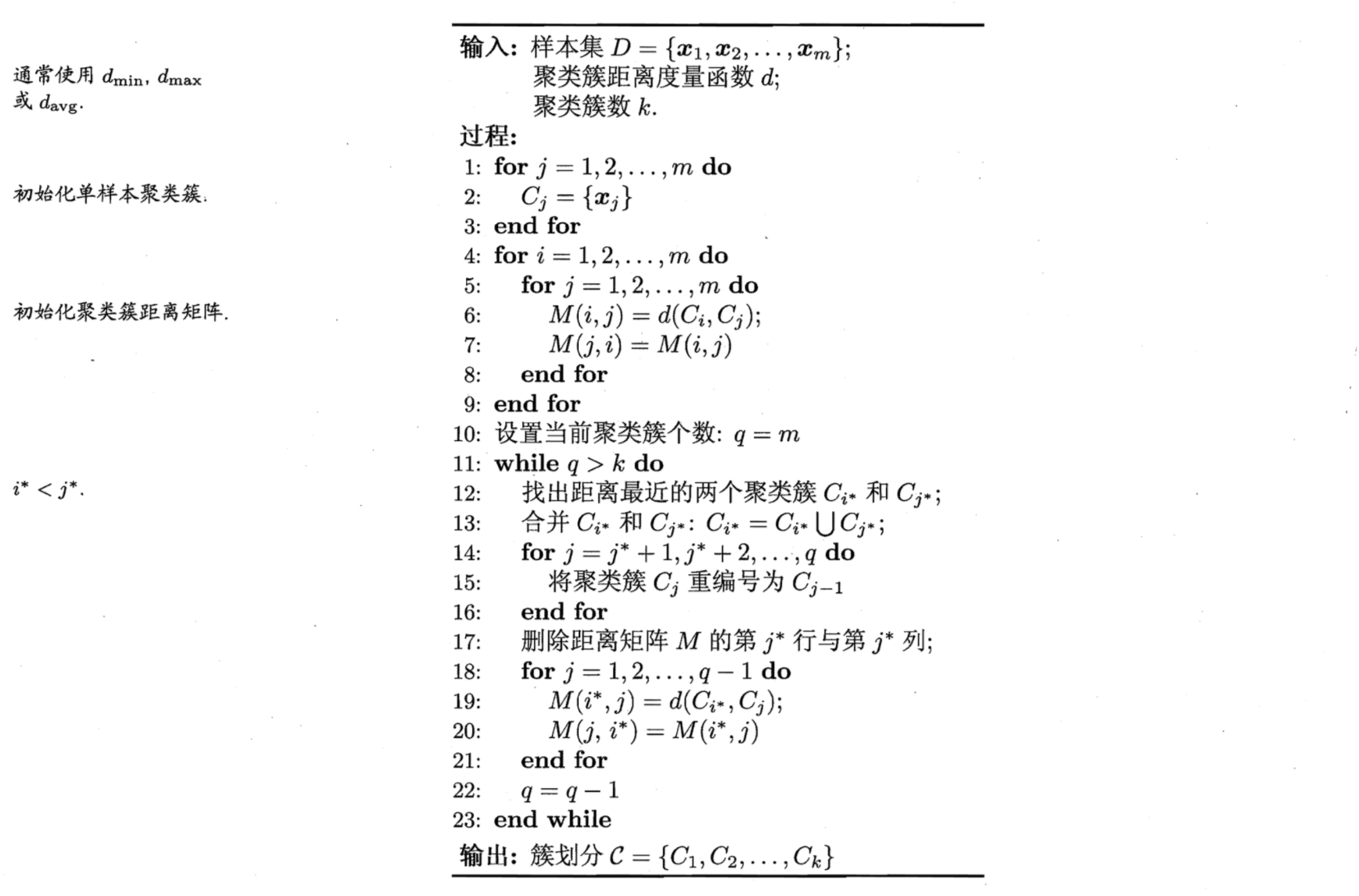图1 凝聚聚类算法

1.2 层次图

下面以单链接为例子，介绍层次聚类的层次图（系统图，dendrogram）。假设我们有以下八个点，使用单链接聚为 3 个类。

首先将每个点设为一个单独的簇类，因此此时有 8 个簇，大于预期 3 个簇，故需要继续进行聚类。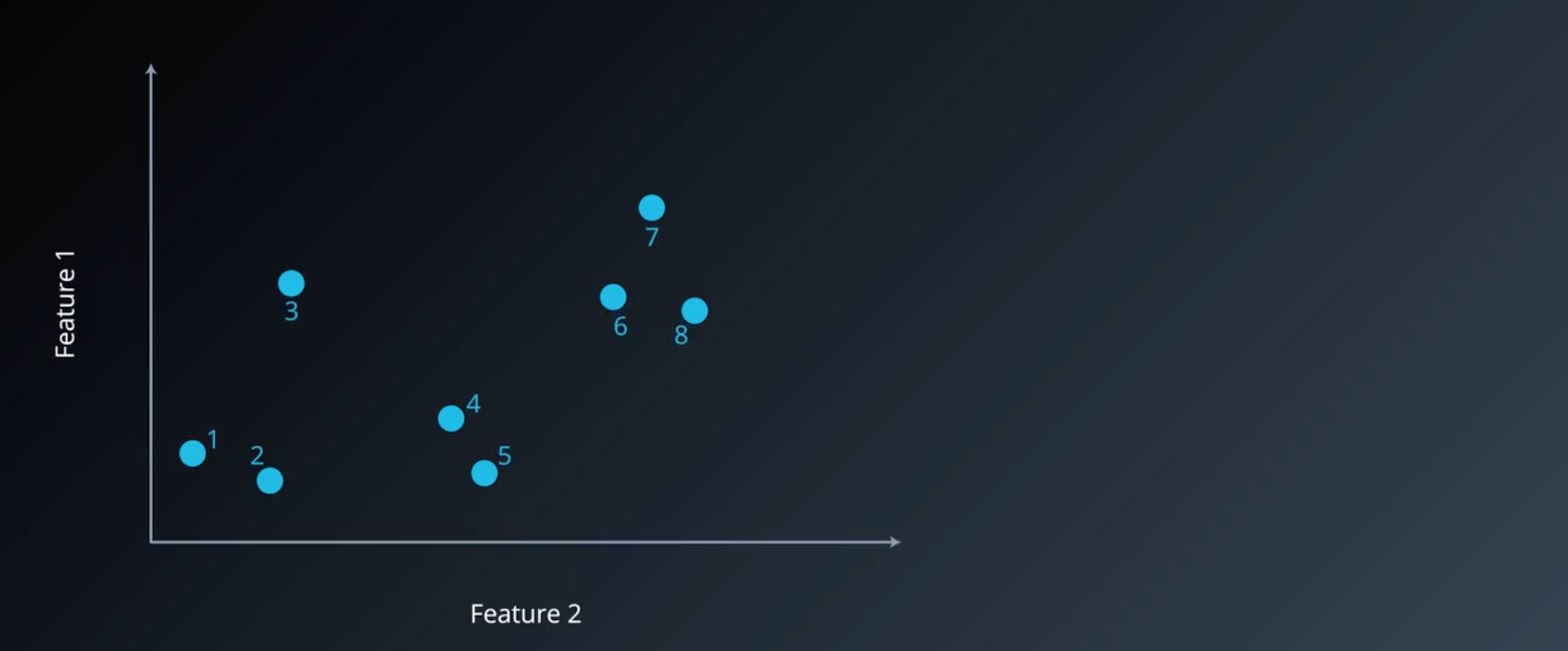图2 初始化聚类簇

因此我们重复算法 11-23 行 3 次，每次将距离最近的两个样本聚为同一簇，对应右图的层次图。此时仍剩下 5 个簇，因此需要继续进行聚类。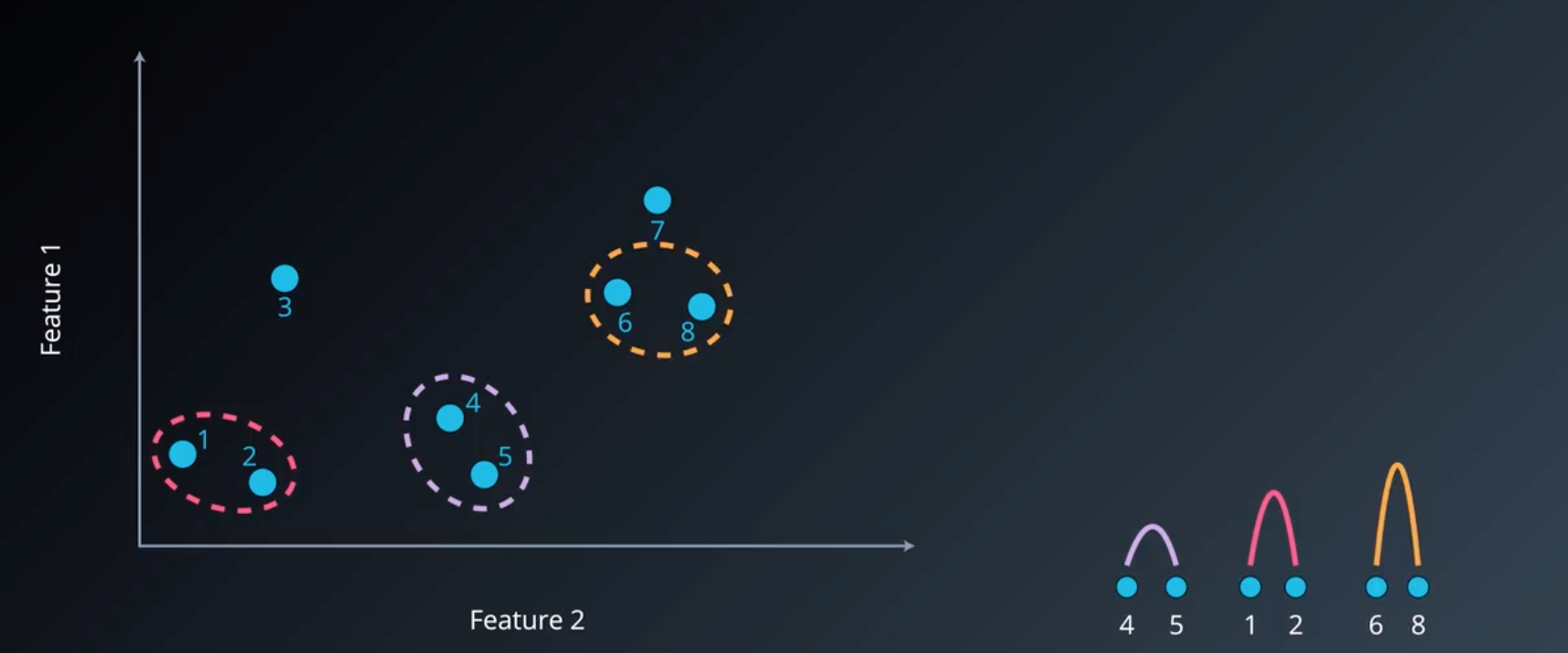图3 5个聚类簇

可以看到，此时样本 7 与簇 {6,8} 的距离（ d i s t m i n = d i s t ( 6 , 7 ) dist_{min}=dist(6,7) ）最近，因此可以将他们聚为一簇，如下图所示：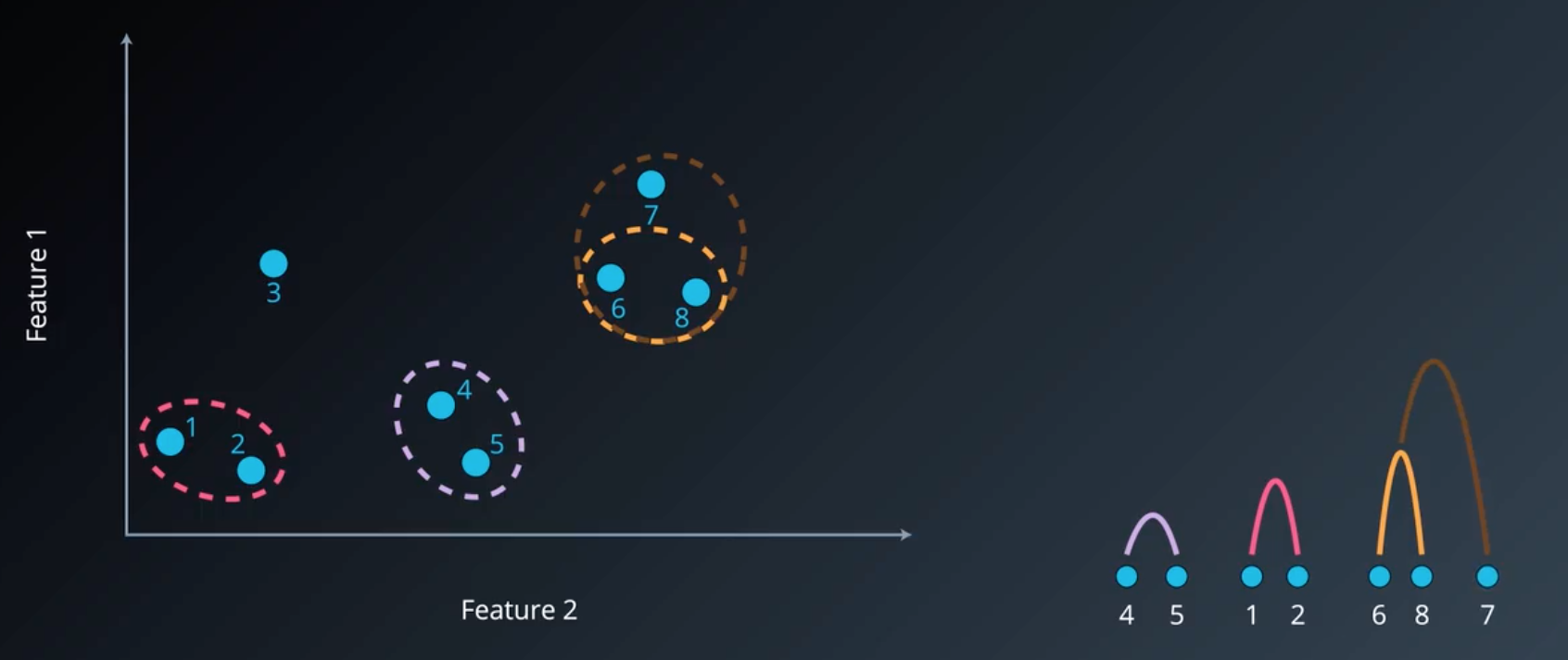图4 4个聚类簇

同理，继续进行聚类，考虑各个簇之间的距离，可以看出，样本 3 与簇 {4,5} 的距离（ d i s t m i n = d i s t ( 3 , 4 ) dist_min=dist(3,4) ）最近，因此有：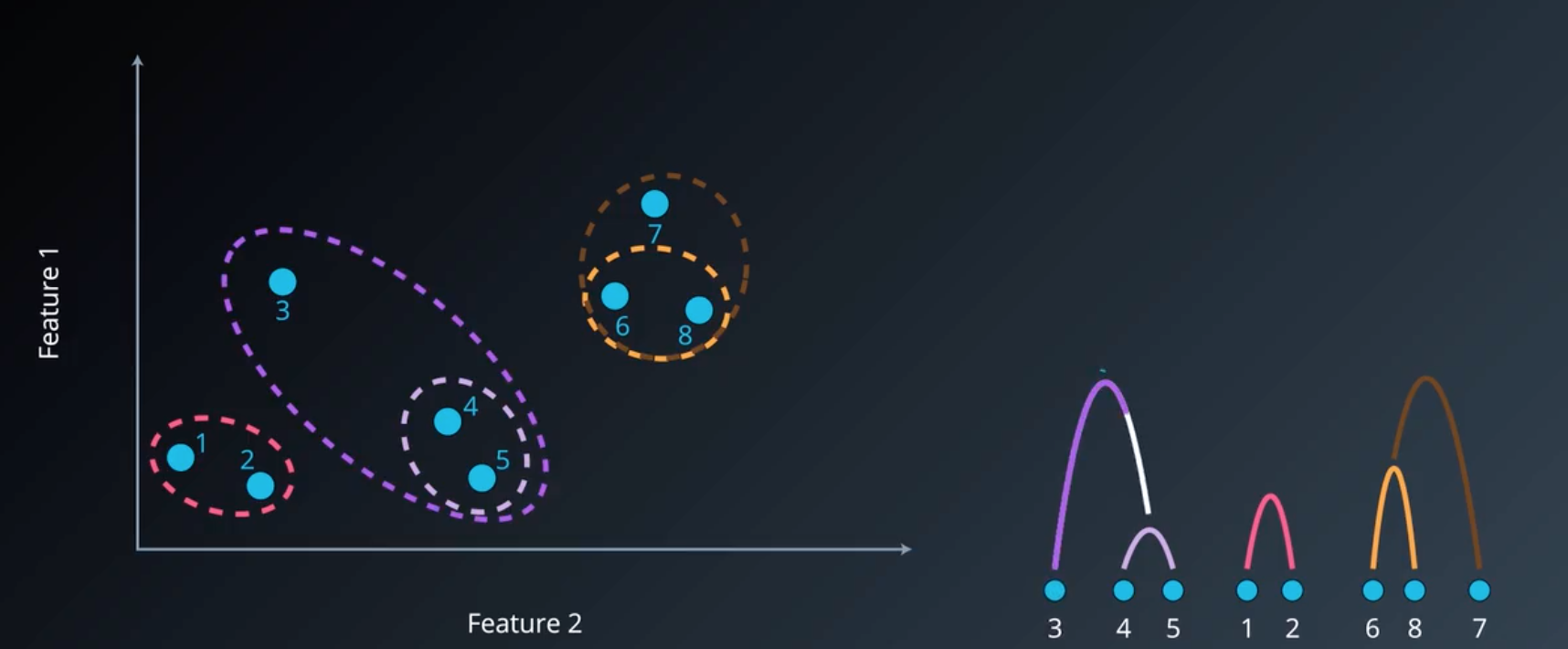图5 3个聚类簇

此时已经达到了我们所要求的聚类簇数，故算法停止，对应的层次图如右方所示。

1.3 不同凝聚算法比较

通过下面图片，我们可以看到各类凝聚算法在不同类型数据上的聚类效果，

需要注意的是，由于单链接和全链接只考虑簇中两个代表性的点，故受噪声和异常点影响大；而单链接容易出现一个簇囊括大多数样本（左下方图），而全链接则比单链接更紧凑些。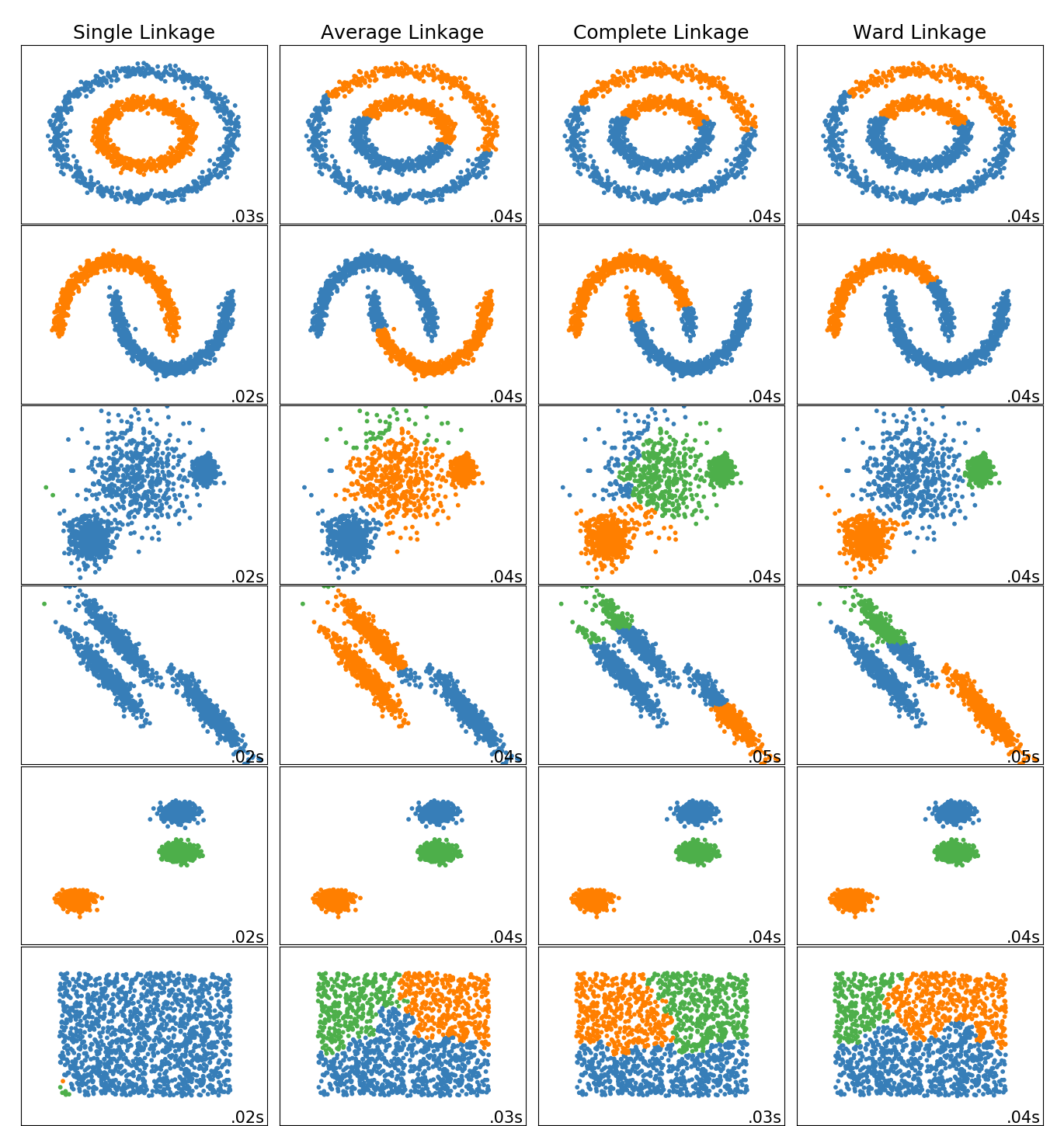图6 不同凝聚算法比较

2. Sklearn 实现

sklearn.cluster.AgglomerativeClustering(n_clusters=2, affinity=‘euclidean’, memory=None, connectivity=None, compute_full_tree=‘auto’, linkage=‘ward’, distance_threshold=None)

n_clusters：聚类簇数

affinity： string or callable, default: “euclidean” 【距离计算参数】

Metric used to compute the linkage. Can be “euclidean”, “l1”, “l2”, “manhattan”, “cosine”, or “precomputed”. If linkage is “ward”, only “euclidean” is accepted. If “precomputed”, a distance matrix (instead of a similarity matrix) is needed as input for the fit method.

linkage： {“ward”, “complete”, “average”, “single”}, optional (default=”ward”)

Which linkage criterion to use. The linkage criterion determines which distance to use between sets of observation. The algorithm will merge the pairs of cluster that minimize this criterion.

• ward minimizes the variance of the clusters being merged.

• average uses the average of the distances of each observation of the two sets.

• complete or maximum linkage uses the maximum distances between all observations of the two sets.

• single uses the minimum of the distances between all observations of the two sets.

2.1 层次图可视化

由于 Sklearn 中并不支持可视化层次图，因此我们使用 Scipy：

from scipy.cluster.hierarchy import dendrogram, ward, single
import matplotlib.pyplot as plt

plt.show

<function matplotlib.pyplot.show(*args, **kw)>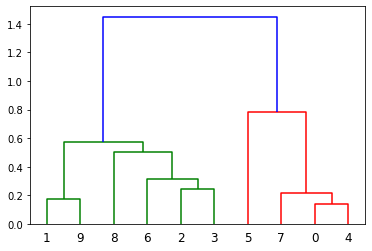图7 层次树可视化

参考文献

 周志华. 机器学习[M]. 北京: 清华大学出版社, 2016: 214.

 Ward J H , Jr. Hierarchical Grouping to Optimize an Objective Function[J]. Journal of the American Statistical Association, 1963, 58(301):236-244.

展开全文机器学习 无监督学习 层次聚类 凝聚聚类 sklearn
• 基本的层次聚类算法matlab实现 简单明了 是我以前上课时记下的笔记内容 代码在15b上实验证实可用
• 聚类算法k-means和层次聚类的java源代码~k-means
• 层次聚类(Hierarchical Clustering)是聚类算法的一种，通过计算不同类别数据点间的相似度来创建一棵有层次的嵌套聚类树。机器学习 层次聚类
• %生成20个随机样本 %屏幕输出Q型聚类结果 %屏幕输出R型聚类结果 包含具体聚类步骤和算法，自写函数体层次聚类
• 程序示例，使用C语言，实现不同数据的分类，包括C均值法和层次聚类法。
• 关于层次聚类(hierarchical clustering)的基本步骤： 1、假设每个样本为一类，计算每个类的距离，也就是相似度 2、把最近的两个合为一新类，这样类别数量就少了一个 3、重新新类与各个旧类(去了那两个合并的类)之间...层次聚类
• 层次聚类(AGNES)算法(Python) 是聚类算法的一种，通过计算不同类别数据点间的相似度来创建一棵有层次的嵌套聚类树。在聚类树中，不同类别的原始数据点是树的最低层，树的顶层是一个聚类的根节点。AGNES是常用的一种...
• 层次聚类算法

2018-03-30 09:10:18
关于层次聚类的一些算法的vvvvvv 懂得而奥迪哥如果该打发额而发阿打发层次聚类
• 凝聚层次聚类层次聚类方法凝聚层次聚类算法原理簇间距离计算方法单链法single全链法complete组平均法 averageward法python代码实现绘制层次聚类树状图一些参考 相关文章： 数据挖掘 | [关联规则] 利用apyori库的关联...聚类 数据挖掘 python 机器学习 大数据
• 层次聚类算法原理及实现

万次阅读 2017-12-21 17:29:32
层次聚类和点分配法聚类。 1.1 点、空间和距离 点集是一种适合于聚类的数据集，每个点都是某空间下的对象。一般意义上，空间只是点的全集，也就是说数据集中的点从该集合中抽样而成。特别地，欧式
• #采用最小距离作为聚类标准 _min_distance=10000 for x1,y1 in cluster1: for x2,y2 in cluster2: _distance=(x1-x2)**2+(y1-y2)**2 if _distance _min_distance=_distance return _distance groups=get_raw...迭代 聚类 python 机器学习
• from scipy.cluster import hierarchy0.层次聚类的概念层次聚类和k-means一样都是很常用的聚类方法。...层次聚类又分为自底而上的聚合层次聚类和自顶而下的分裂层次聚类。0.1 聚合层次聚类每一个点初始为1类，得到N(...
• 起步层次聚类( Hierarchical Clustering )是聚类算法的一种，通过计算不同类别的相似度类创建一个有层次的嵌套的树。层次聚类算法介绍假设有 n 个待聚类的样本，对于层次聚类算法，它的步骤是：步骤一：(初始化)将每...
• Sklearn实现层次聚类

千次阅读 2021-01-13 17:52:29
层次聚类(Hierarchical clustering)代表着一类的聚类算法，这种类别的算法通过不断的合并或者分割内置聚类来构建最终聚类。 聚类的层次可以被表示成树（或者树形图(dendrogram)）。树根是拥有所有样本的唯一聚类，...聚类 机器学习 sklearn
• 四、（2） 文本层次聚类

千次阅读 2019-05-18 11:30:35
四、(1) 层次聚类 层次聚类方法的基本思想是：通过某种相似性测度计算节点之间的相似性，并按相似度由高到低排序，逐步重新连接个节点。该方法的优点是可随时停止划分，主要步骤如下： （1）移除网络中的所有边，...
• 层次聚类(Hierarchical Clustering)是聚类算法的一种，通过计算不同类别数据点间的相似度来创建一棵有层次的嵌套聚类树。在聚类树中，不同类别的原始数据点是树的最低层，树的顶层是一个聚类的根节点。创建聚类树有...
• 基于层次的聚类方法 简介 基于层次的聚类方法 概念 聚合层次聚类 图示 划分层次聚类 图示 基于层次的聚类方法 切割点选取 ...基于层次聚类 ( 聚合层次聚类 ) 算法终止条件 族半径 计算公式 基于层次聚类总结聚类 基于层次聚类 数据挖掘 机器学习
• 层次聚类算法及其实现

千次阅读 2016-03-10 19:57:41
层次聚类算法分为合并算法和分裂算法。合并算法会在每一步减少聚类中心的数量，聚类产生的结果来自前一步的两个聚类的合并；分裂算法与合并算法原理相反，在每一步增加聚类的数量，每一步聚类产生的结果都将是前一步......